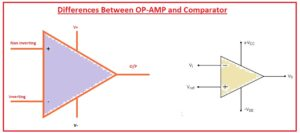Hello readers welcome to the new post. In this post, we will learn Differences Between OP-AMP and Comparator. These 2 devices are the part of differential amplifier family and there are common features are inputs and output terminals. There are symbolic representation is also same. If we do a comparison between them come to know that there is an open circuit collector at the comparator and at the operational amplifier there is NPN and PNP configuration.

Here we discuss their different parameters and finds their common differences. So let get started.

## Differences Between Operational Amplifier and Comparator

What is Operational Amplifier

• The operational amplifier is an amplifier having a large gain coupled to DC also comes with differential inputs means positive and negative.
• There is a single input terminal it has. Its output volts have a one lac time larger value than the inputs volts.
• There is linear, arithmetic and nonlinear function in the analog computer are done by this module.
• Its versatile nature makes it useful in analog modules.
• It has two main types first one is the inverting and the second one is non inverting operational amplifier.
• The output, gain value input, and value of output impedance can be measured through use of the negative feedback function.
• It is employed at industry, electronic modules, and different instruments used in the laboratory.
• Its prices are very less but some hybrid OP amp has a larger cost due to some extra features.Introduction to Inverting Amplifier

• his category of the amplifier provides the output at an angle of one-eight degrees than input. IF a positive value of supply is provided to this circuitry output of the circuitry will be negative.
• There are one-eighty degrees of phase difference between input and output.
• Input is given at the non-inverting terminal or negative of circuitry.
• Its polarity is negative than another amplifier.
• It’s gaining the ratio of resistance at output and input.

What is a Non-Inverting Amplifier

• The output of this amplifier is in phase to the input given at this circuitry.
• Its gain polarity is positive.
• Gin is the sum of one and the ratio of resistance
• There is zero phase difference between input and output.
• Its input is given at the positive terminal of circuitry.
• Its output has noninverted behavior than inverting amplifier.

### What is Comparator

• It is such a device that generates the output after making the comparison between the applied voltage at 2 terminals.
• The output at the terminals indicates which input has a high value or less.
• It has 2 input terminals and a single output where the comparison of 2 inputs is given.
• It main types are listed here.
• Mechanical Comparator
• Electrical-Electronic Comparator
• Pneumatic Comparator
• Mechanical-Optical Comparator
• Reed Type Comparator

That is the detailed post Differences Between OP-AMP and Comparator. If you havd any further query ask in comments. Thanks for reading have a good day see you in next post.